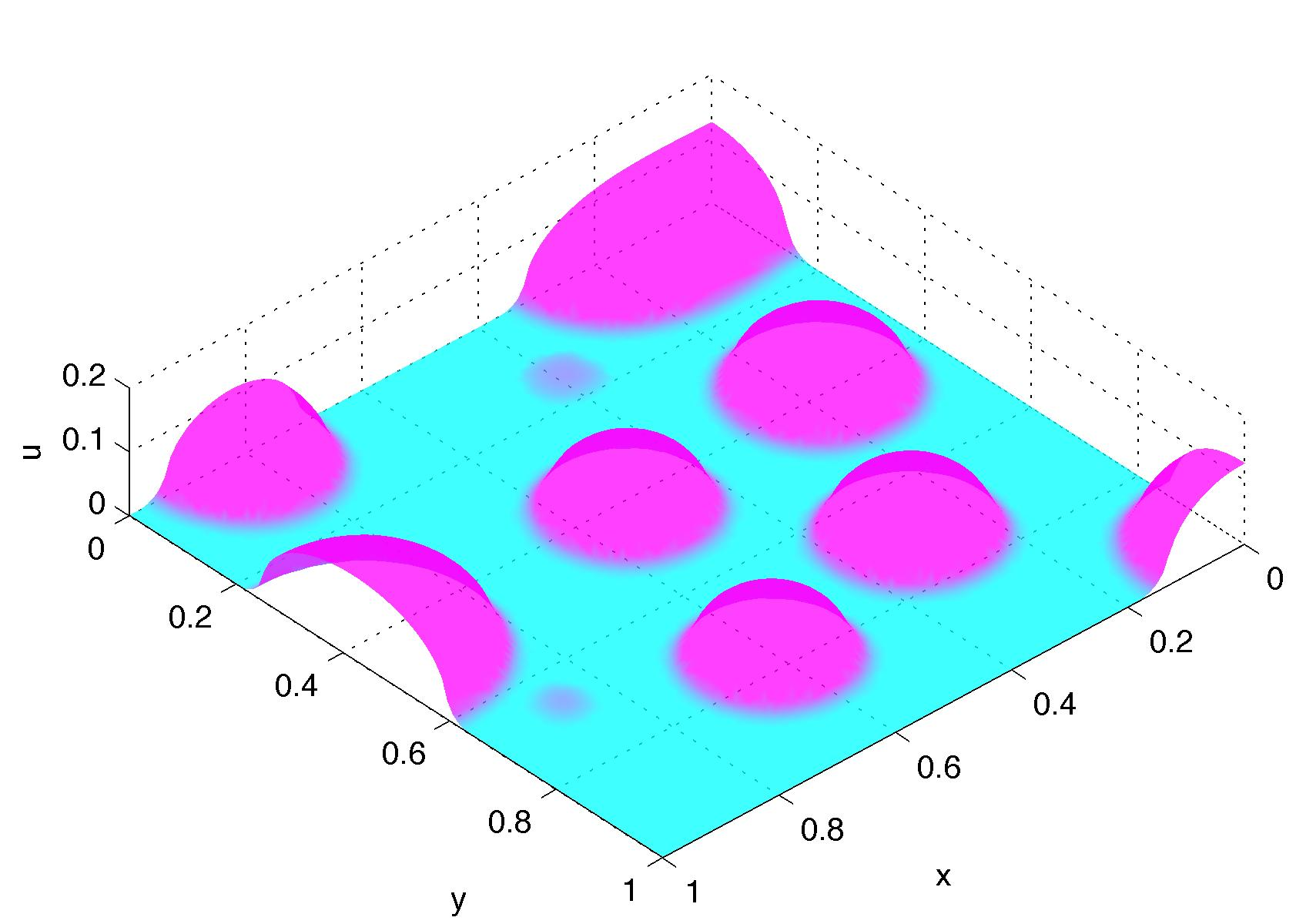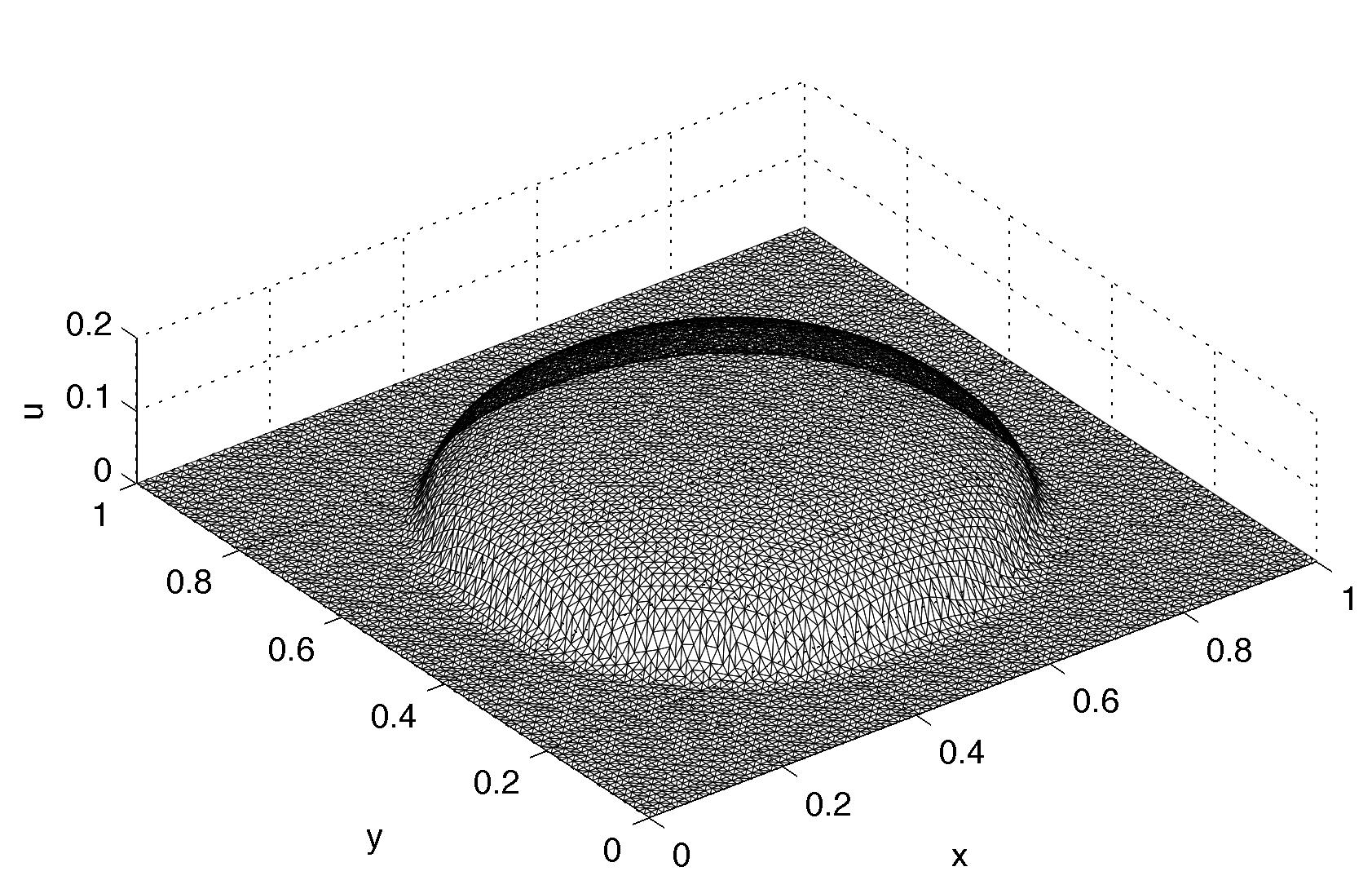##Research

Droplet and bubble motions

Droplets and bubble motions present a recurring topic of the natural sciences. This research is approached in the framework of free boundary problems, utilizing variational techniques. We focus on the dynamical behavior of such objects, both analytically and computationally, while aiming to develop mathematical models for use in applications.

Another key aspect of our research involves developing variational methods (numerical methods) for computing solutions to the target equations. Using knowledge gained through analytical and computational investigations, we want to understand the underlying structure of droplet condensation/evaporation, coalescence/separation, and the role of surface tension in governing the droplet motions (contact angle dynamics). Our investigations have centered on a model equation for describing the scalar motion of droplets and bubbles. The derivation is based on variational principles, formulated as the stationary point of an action integral (together with a free boundary condition that governs the motion of the droplet on its perimeter).We are also investigating our model equation by means of constructing numerical methods for its approximation. Our numerical methods are the computational realization of so-called minimizing movements, that are also used in the mathematical analysis of the model equation. We have found that the variational nature of this approach allows one to easily (automatically) control the volume, contact angles, and to determine the free boundary of each droplet, and that the qualitative behavior seems promising.

The variational formulation gives on a simple way to express and control (both mathematically and computationally) the motion of our droplets and from problems related to droplets on a windshield to the nano-droplet delivery of medicines, we expect that further research will see several applications in industry. Hyperbolic free boundary problems are a completely new area of research in the mathematical sciences and we expect that further research into these objects will put their study on a solid foundation, while, at the same time, opening the doorway into new areas for their application.